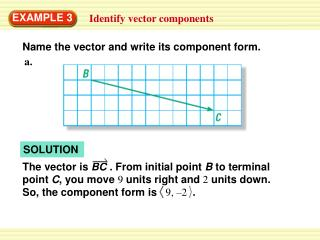DownloadDownload PresentationEXAMPLE 3

# EXAMPLE 3

Télécharger la présentation## EXAMPLE 3

- - - - - - - - - - - - - - - - - - - - - - - - - - - E N D - - - - - - - - - - - - - - - - - - - - - - - - - - -
##### Presentation Transcript

1. a. The vector is BC . From initial point B to terminal point C, you move 9 units right and 2 units down. So, the component form is 9, –2 . EXAMPLE 3 Identify vector components Name the vector and write its component form. SOLUTION

2. b. The vector is ST . From initial point S to terminal point T, you move 8 units left and 0 units vertically. The component form is –8, 0 . EXAMPLE 3 Identify vector components Name the vector and write its component form. SOLUTION

3. First, graph ∆ABC. Use 5, –1 to move each vertex 5 units to the right and 1 unit down. Label the image vertices. Draw ∆ A′B′C′. Notice that the vectors drawn from preimage to image vertices are parallel. EXAMPLE 4 Use a vector to translate a figure The vertices of ∆ABC are A(0, 3), B(2, 4), and C(1, 0). Translate∆ABC using the vector 5, –1 . SOLUTION

4. SOLUTION The vector is RS . From initial point R to terminal point S, you move 5 units right and 0 units vertically. The component form is 5, 0 . for Examples 3 and 4 GUIDED PRACTICE Name the vector and write its component form. 4.

5. The vector is TX . From initial point T to terminal point S, you move 0 units horizontally and 3 units up. The component form is 0, 3 . for Examples 3 and 4 GUIDED PRACTICE 5. Name the vector and write its component form. SOLUTION

6. The vector is BK . From initial point B to terminal point K, you move 5 units left and 2 units up. So, the component form is –5 , 2 . for Examples 3 and 4 GUIDED PRACTICE Name the vector and write its component form. 6. SOLUTION

7. for Examples 3 and 4 GUIDED PRACTICE The vertices of ∆LMN are L(2, 2), M(5, 3), and N(9, 1). Translate ∆LMN using the vector –2, 6 . 7. SOLUTION Find the translation of each vertex by subtracting 2 from its x-coordinate and adding 6 to its y-coordinate. (x, y) → (x – 2, y + 6) L(2, 2) → L′(0, 8) M(5, 3) → M′(3, 9) N(9, 1) → N′(7, 7)